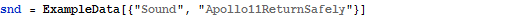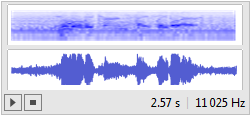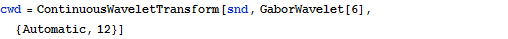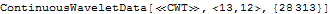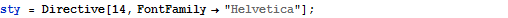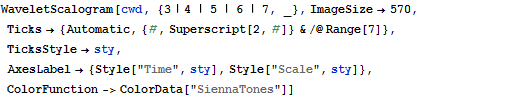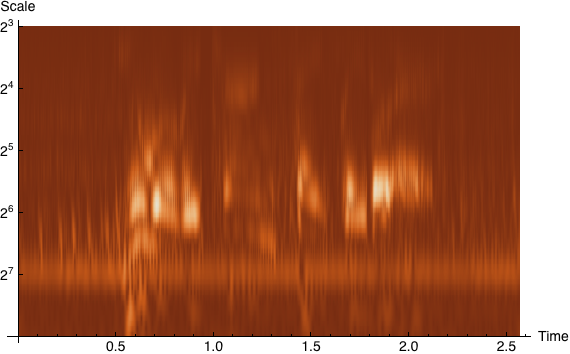# Wavelet Analysis

With Mathematica 8, a complete system of wavelet methods is introduced in Mathematica.
Use discrete or continuous high-performance wavelet analyses for thresholding and visualization in any number of dimensions.

• Represention of many new discrete wavelet functions
• Represention of many new continuous wavelet functions
• Signal and image analysis

The user can transform his data in multiple wavelet bases, wavelet packet bases, or trigonometic bases, and perform inverse transforms in one or two dimensions. After that, the transform can be represented in a time-frequency space, and several different bases. Also, boundary values can be selected. Compressing and denoising of data prove to be surprisingly simple processes, which can be performed by using the integrated functions of the wavelet collection.

## Continuous Wavelet Families

Show the wavelet function () for different continuous wavelet families.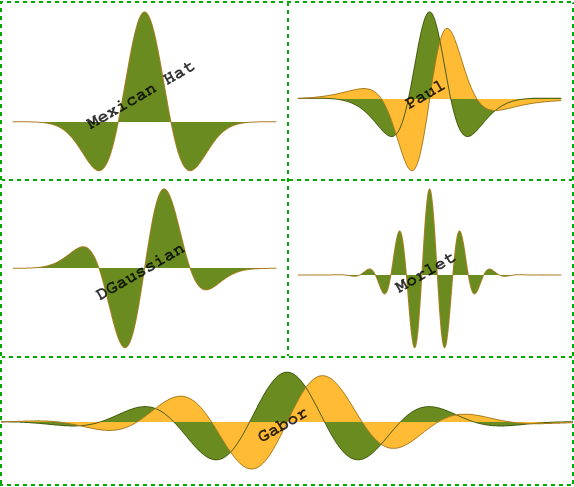## Discrete Wavelet Families

Show the scaling function () and wavelet function () for several discrete wavelet families.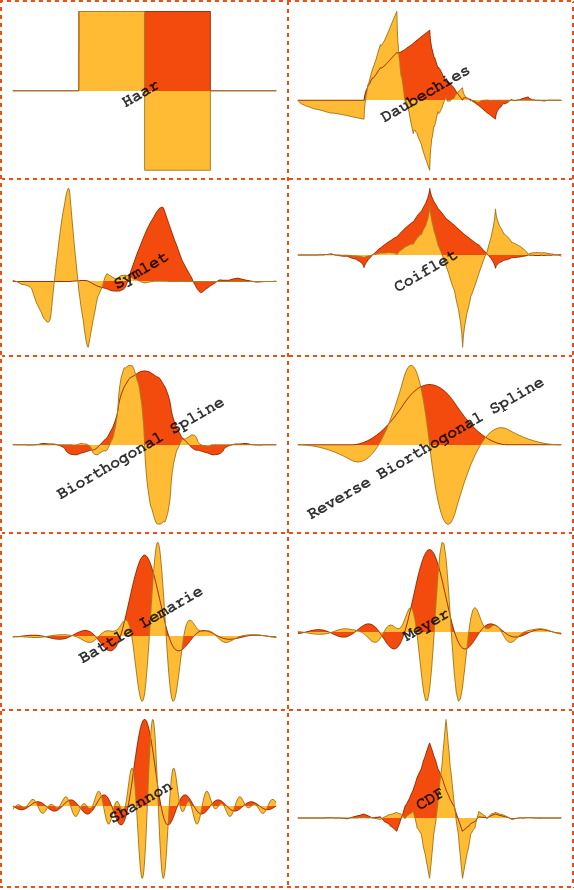## Get Properties for Wavelet Families

Directly get the scaling function (), wavelet function (), and filter coefficients for each wavelet family, or use them to specify a wavelet transform.

To show Mathematica code, please click "plus".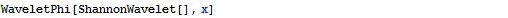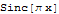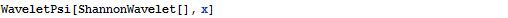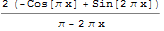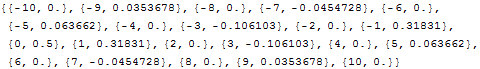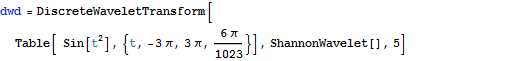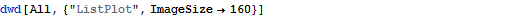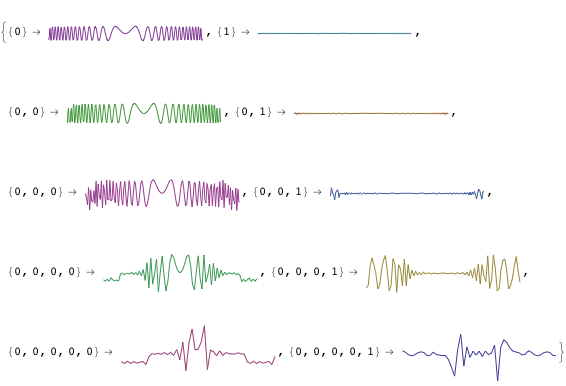## Discrete Wavelet Transform (DWT)

Perform a "DiscreteWaveletTransform".

To show Mathematica code, please click "plus".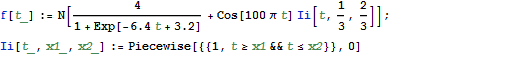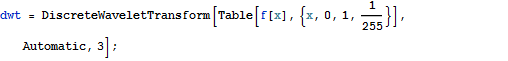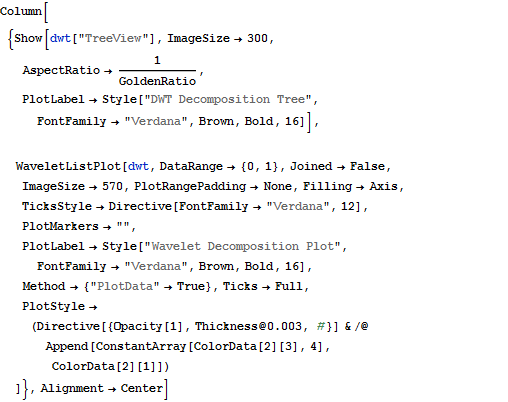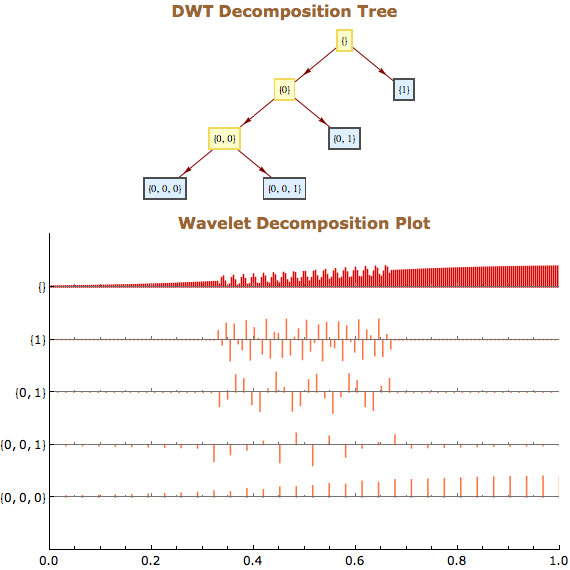## Lifting Wavelet Transform (LWT)

Perform a "LiftingWaveletTransformation".

To show Mathematica code, please click "plus".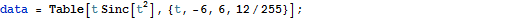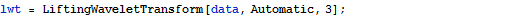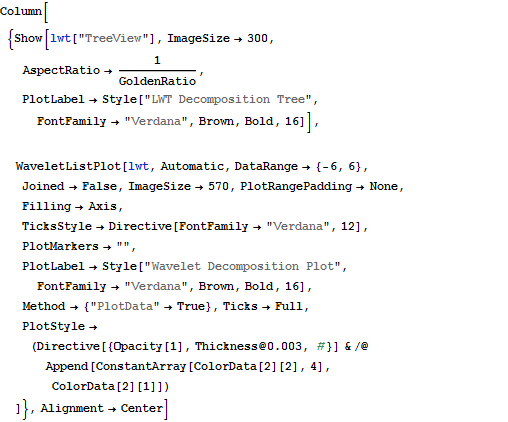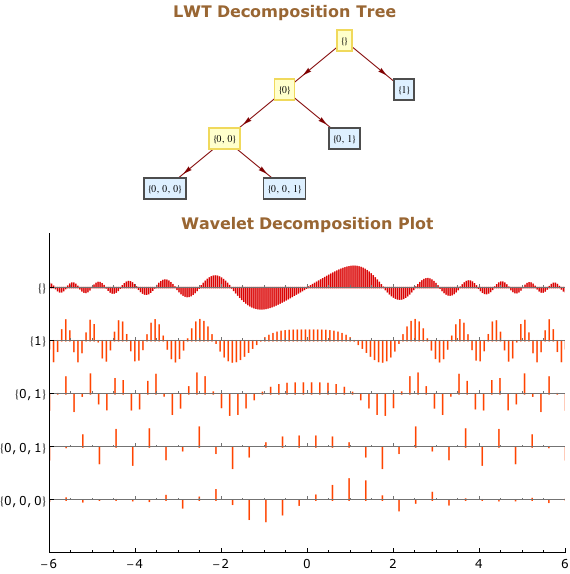## Discrete Wavelet Packet Transform (DWPT)

Perform a "DiscreteWaveletPacketTransform".

To show Mathematica code, please click "plus".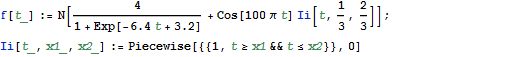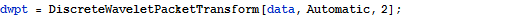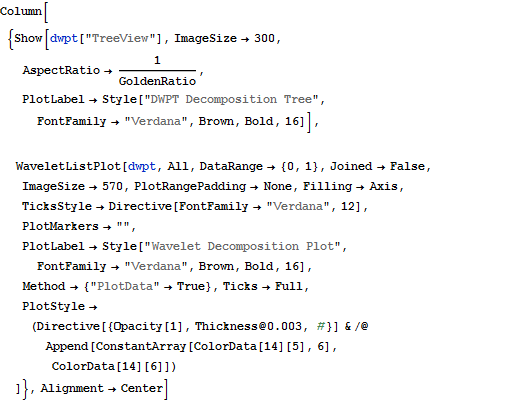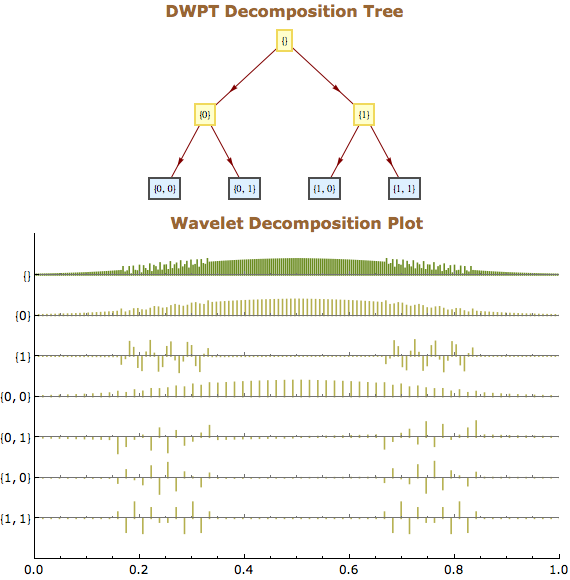## Stationary Wavelet Packet Transform (SWPT)

Perform a "StationaryWaveletPacketTransform".

To show Mathematica code, please click "plus".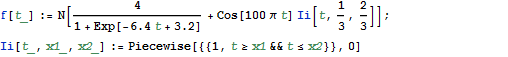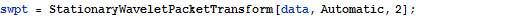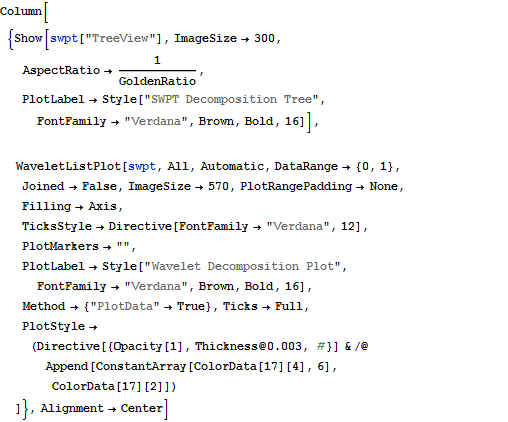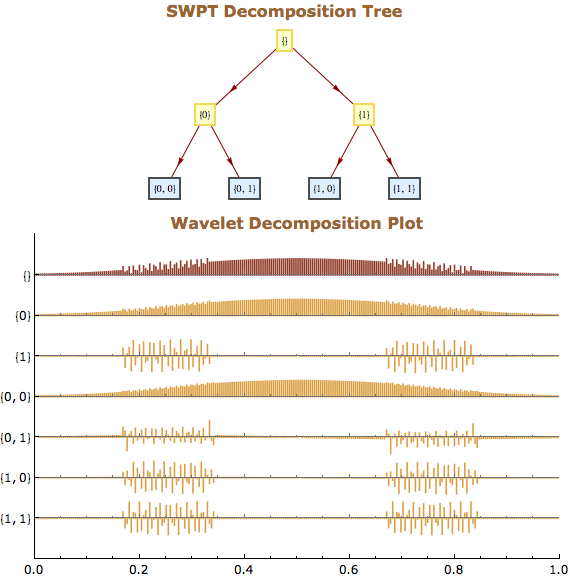## Compare Discrete Wavelet Transforms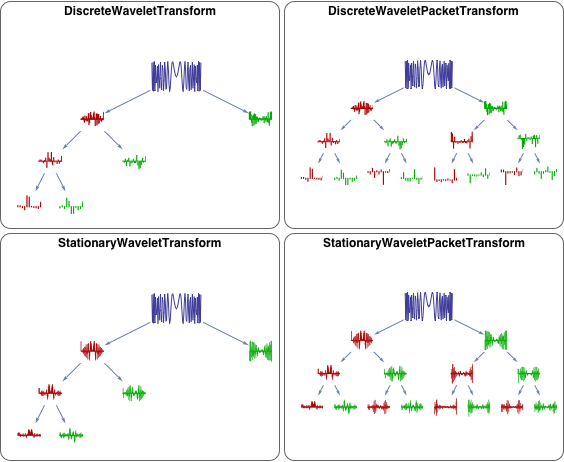## Transform Images

Perform wavelet packet transforms directly on images.

To show Mathematica code, please click "plus".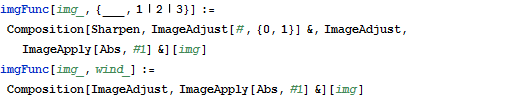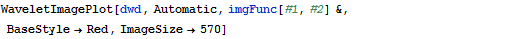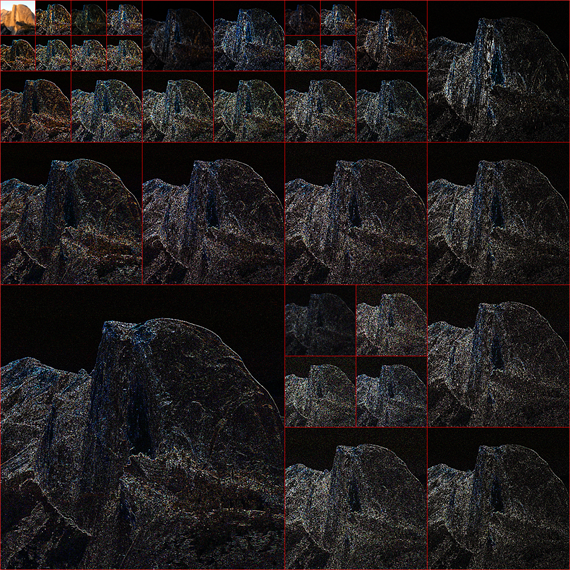## Wavelet Image Fusion

Blend two images.

To show Mathematica code, please click "plus".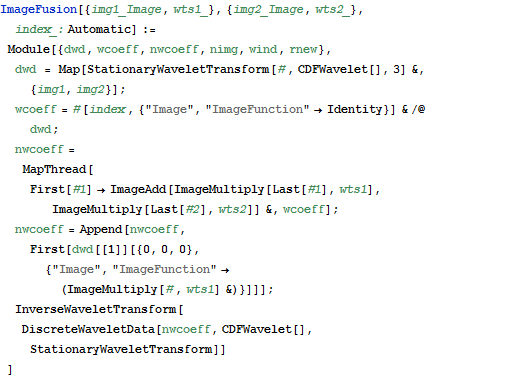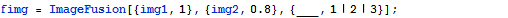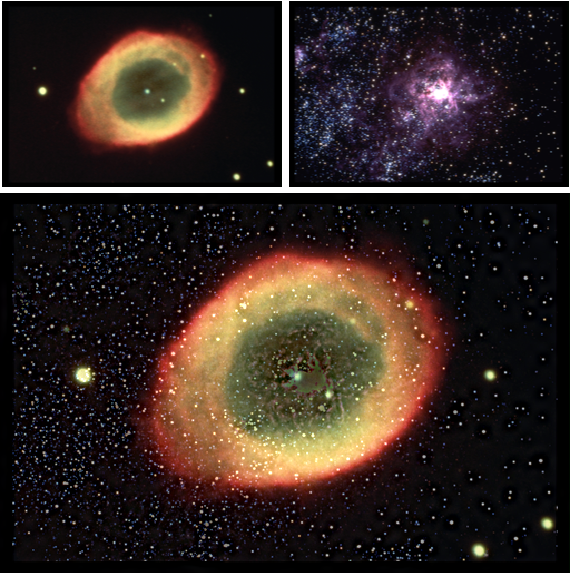## Visualize Scalograms

To show Mathematica code, please click "plus".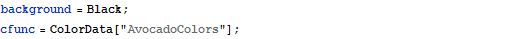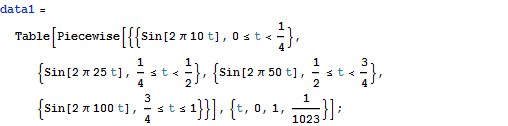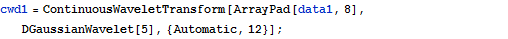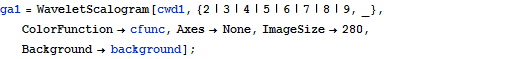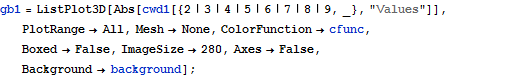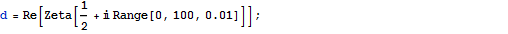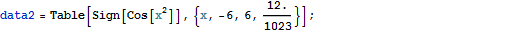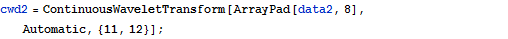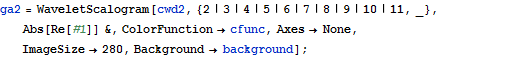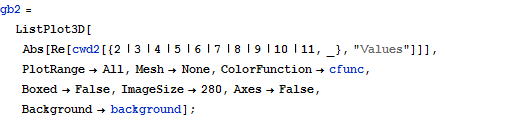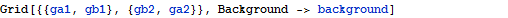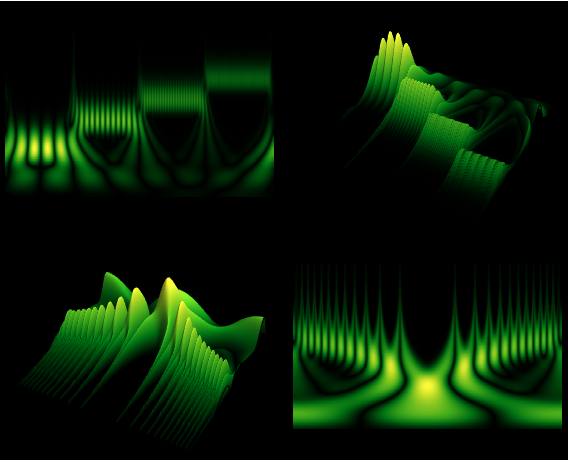## Transform Sound

Directly analyze a sound using continuous wavelet transforms.

To show Mathematica code, please click "plus".